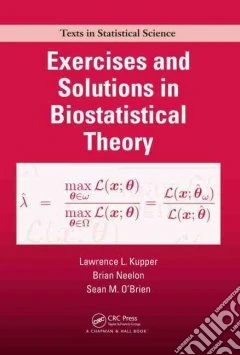ricerca
avanzata

# Exercises and Solutions in Biostatistical Theory

## Un libro in lingua di Kupper Lawrence L.Neelon Brian H.O'brien Sean M. edito da Chapman & Hall, 2010

• € 44,70
• Il prezzo è variabile in funzione del cambio della valuta d’origineExercises and Solutions in Biostatistical Theory presents theoretical statistical concepts, numerous exercises, and detailed solutions that span topics from basic probability to statistical inference. The text links theoretical biostatistical principles to real-world situations, including some of the authors' own biostatistical work that has addressed complicated design and analysis issues in the health sciences.

This classroom-tested material is arranged sequentially starting with a chapter on basic probability theory, followed by chapters on univariate distribution theory and multivariate distribution theory. The last two chapters on statistical inference cover estimation theory and hypothesis testing theory. Each chapter begins with an in-depth introduction that summarizes the biostatistical principles needed to help solve the exercises. Exercises range in level of difficulty from fairly basic to more challenging (identified with asterisks).

Features

Contains many exercises of varying difficulty often drawn from real-life applications, especially in the health sciences

Explains the theoretical biostatistical principles needed to solve the exercises

Includes detailed solutions to all exercises

Presents useful mathematical results in an appendix

By working through the exercises and detailed solutions in this book, readers will develop a deep understanding of the principles of biostatistical theory. The text shows how the biostatistical theory is effectively used to address important biostatistical issues in a variety of real-world settings. Mastering the theoretical biostatistical principles described in the book will prepare readers for successful study of higher-level statistical theory and will help them become better biostatisticians.